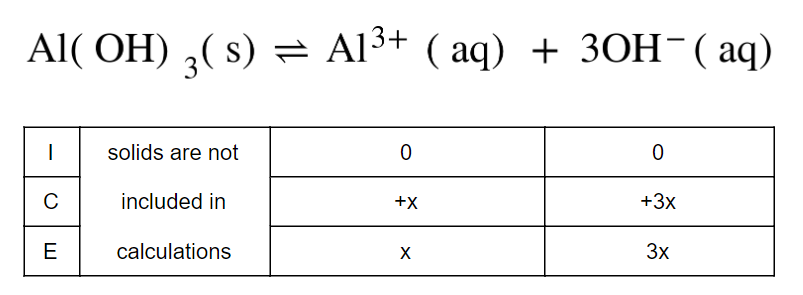Problem: What is the Ksp of Al(OH)3 if the concentration of OH– in a saturated solution of Al(OH)3 is 1.6 × 10–8 M?a. 2.6 x 10-16 b. 1.6 x 10-23c. 1.8 x 10-30 d. 2.2 x 10-32

FREE Expert Solution
91% (133 ratings)
FREE Expert Solution

Setup an ICE table to determine the concentration of [OH-]

Dissociation of  Al(OH)3  will appear as:91% (133 ratings)
Problem Details

What is the Ksp of Al(OH)3 if the concentration of OH in a saturated solution of Al(OH)3 is 1.6 × 108 M?

a. 2.6 x 10-16

b. 1.6 x 10-23

c. 1.8 x 10-30

d. 2.2 x 10-32

What scientific concept do you need to know in order to solve this problem?

Our tutors have indicated that to solve this problem you will need to apply the Ksp concept. You can view video lessons to learn Ksp Or if you need more Ksp practice, you can also practice Ksp practice problems .

What is the difficulty of this problem?

Our tutors rated the difficulty of What is the Ksp of Al(OH)3 if the concentration of OH– in a ... as medium difficulty.

How long does this problem take to solve?

Our expert Chemistry tutor, Dasha took 4 minutes to solve this problem. You can follow their steps in the video explanation above.

What professor is this problem relevant for?

Based on our data, we think this problem is relevant for Professor Tang's class at USF.